Boards are fundraising to help the people of Ukraine via the Red Cross at this horrific time. Please donate and share if you can, you will find the link here. Many thanks.

# ** LC Applied Maths 2014 - Before and after discussion **

• mcratsix wrote: »
Ewwww q9. Why not q3? That's like easiest q on the entire exam, in my opinion.

Can't do trig for the life of me. It's grand in maths, but the trig in applied maths, particularly question 3, is horrendous. I like question 9, the pressures and relative densities of mixtures and stuff comes pretty easily to me. Our teacher didn't do the circular motion or inertia questions, so we're limited to 1, 2, 3, 4, 5, 7, 9 & 10, and 7 is almost invariably the hardest on the paper.

• Can't do trig for the life of me. It's grand in maths, but the trig in applied maths, particularly question 3, is horrendous. I like question 9, the pressures and relative densities of mixtures and stuff comes pretty easily to me. Our teacher didn't do the circular motion or inertia questions, so we're limited to 1, 2, 3, 4, 5, 7, 9 & 10, and 7 is almost invariably the hardest on the paper.

Leaving out moments of inertia is a big mistake on your teachers part, it's quite simple usually.

• Do the proofs for 8a have to be same as marking scheme or can they be from the book?

• rocco123 wrote: »
Do the proofs for 8a have to be same as marking scheme or can they be from the book?

From the book is fine according to my teacher

• @mcratsix I thanked one of your posts, but I thoroughly disagree with your sentiments regarding Statics!

@TheBegotten I agree that some Q3s have had fiendish trig manipulations.
Last I checked it wasn't an originality test! If it was I'd be doing Hydrostatics. Has it really been that long for a wedge? I want one sooo badly! We didn't study RMB so I wouldn't know how easy it is, which Q is it normally on the paper?

I really need an A1. Don't know if I can pull it off. 2 days free before the exam though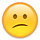This could be a rumour, but I heard from a guy who got 100% on every question that AM is the only subject apart from English that uses the PCLM marking scheme: only the ten percent for "mechanics" actually goes towards how "correct" your answer is. Therefore, I'd encourge that you try to be really original! Try things the corrector will neve have seen before, like da Vinci-esque mirror writing. Let me know how you get on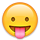Now that I think about it, as well as RBM (Q8), RV can be pretty handy. None of the topics is especially difficult, but some of the q's can be, so when I say RBM is one of the easiest, I mean the questions have been pretty standard (plus the free twenty marks for the easiest-to-learn proofs ever to have been prescribed!!).

There was a point in the exam when I had to decide whether to abandon a q or keep hammering. I chose the former and ended up getting an A1. Having taken ages to get the answer on the way home afterwards, I guarantee you I would have got around a C if I hadn't moved on. I suspect loads of students capable of getting an A (and let's face it, AM more than perhaps any other subject, has loads of students who could get an A depending on how well the exam goes) fail to get one because of getting bogged down (actually, now that I think about it, that theory falls down considering how few cover more than seven topics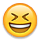).

Rmember that most marks go for setting the q up. Carefully review every answer, cause sometimes correcting a small mistake can move you up a level in the MS resulting in your getting a chunk of marks. It's a complete disaster if you don't give every part a decent attempt. Rememer that a-parts are often equal to b-parts in term of marks. At this stage, I'd be going through past papers and answering them in my head.

Erm...anything else? Oh, sometimes bringing an extra teddy can pay dividends!

Good luck everyone. And don't feel any pressure because of the fact that I still judge people in college on the basis of their AM result! What?! I can't help it• Anyone else the only person doing Applied Maths in their school? Will be a bit awkward in the exam hall

Last year my supervisor offered me tea when it was just me and him in the exam hall.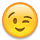• What are the chances of them asking a topic in a different question to the norm?

In 2002, Simple Harmonic motion came up in Q4(a). Has this happened elsewhere? :eek:

• What are the chances of them asking a topic in a different question to the norm?

In 2002, Simple Harmonic motion came up in Q4(a). Has this happened elsewhere? :eek:

There would be uproar if they do something like SHM in Q4 but I can see them linking similar topics. Putting in an impact or a bouncing projectile into Q3 would make sense.
They know most students don't cover the whole syllabus so I can't see them destroying the bell curve just to test us.

• What are the chances of them asking a topic in a different question to the norm?

In 2002, Simple Harmonic motion came up in Q4(a). Has this happened elsewhere? :eek:

Yeah it happens from time to time, just after doing a question in relative velocity that involved collisions.

• This SHM question (2004 Q4(a)) had nowt to do with Connected Particles though, hence my panic. I've spent an hour learning the basics of SHM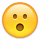• This SHM question (2004 Q4(a)) had nowt to do with Connected Particles though, hence my panic. I've spent an hour learning the basics of SHMUnless you're going to do SHM I don't think it's worth it. If they can randomly put SHM into a question, why not statics or moments of inertia? This is why it's good to have a spare question just in case they do through a curve ball like this.

• Does anyone have results for Mocks questions? (1,2,3,4,5,10)
I'm not sure if it's DEB or Examcraft, so I'll write out question 1.

Q1. a) The maximum acceleration of a body is 9m/s^2 and its maximum ratardation is 3m/s^2. What is the shortest time in which the body can travel....
b) A car P start from a point O with initial velocity of 6m/s and then travels with a uniform acceleration of 3m/s^2. Three seconds later...

Thanks

• Nicke, that's Examcraft. I haven't got the solutions though. Sorry.

• Nicke011 wrote: »
Does anyone have results for Mocks questions? (1,2,3,4,5,10)
I'm not sure if it's DEB or Examcraft, so I'll write out question 1.

Q1. a) The maximum acceleration of a body is 9m/s^2 and its maximum ratardation is 3m/s^2. What is the shortest time in which the body can travel....
b) A car P start from a point O with initial velocity of 6m/s and then travels with a uniform acceleration of 3m/s^2. Three seconds later...

Thanks

That's examcraft, I have my attempt at it with my teachers corrections, I'm not sure if it would just be confusing though?

• For the Wedges, can someone explain to me why the accelerations of the particles relative to the wedge are mthe way they are. i.e for the acc perpendicular to the slope. it is mgcos(theta)-R=m(asin(theta)). I know that's how it's done but I don't actually see where the m(asin(theta)) part comes from.

• Chonaic17 wrote: »
For the Wedges, can someone explain to me why the accelerations of the particles relative to the wedge are mthe way they are. i.e for the acc perpendicular to the slope. it is mgcos(theta)-R=m(asin(theta)). I know that's how it's done but I don't actually see where the m(asin(theta)) part comes from.

Its Newtons Second Law, F =ma
This is a little tricky to explain without a diagram but basically you splitting the acceleration up into horizontal and vertical components
I assume we are looking at vertical components here due to R being used in the equation, so the force, F = mgCos(theta) - R
Similar to how you split up the weight of the object, mg, relative to the wedge, you must do the same with the acceleration.
The acceleration in the vertical direction will be given by aSin(theta), so by Newtons Second Law you get mgCos(theta) - R = m(aSin(theta).

• Ompala wrote: »
Its Newtons Second Law, F =ma
This is a little tricky to explain without a diagram but basically you splitting the acceleration up into horizontal and vertical components
I assume we are looking at vertical components here due to R being used in the equation, so the force, F = mgCos(theta) - R
Similar to how you split up the weight of the object, mg, relative to the wedge, you must do the same with the acceleration.
The acceleration in the vertical direction will be given by aSin(theta), so by Newtons Second Law you get mgCos(theta) - R = m(aSin(theta).

Oh wow it just hit me there as I read this, thanks a lot that makes perfect sense.• Ompala wrote: »
Its Newtons Second Law, F =ma
This is a little tricky to explain without a diagram but basically you splitting the acceleration up into horizontal and vertical components
I assume we are looking at vertical components here due to R being used in the equation, so the force, F = mgCos(theta) - R
Similar to how you split up the weight of the object, mg, relative to the wedge, you must do the same with the acceleration.
The acceleration in the vertical direction will be given by aSin(theta), so by Newtons Second Law you get mgCos(theta) - R = m(aSin(theta).

The mgcos(theta) is the weight of the particle acting perpendicular to the slop, the R is the reaction of the wedge pushing up on the particle. Since the particle is sliding down the wedge, we know that the force acting down must be greater than the force acting up, hence we get mgcos(theta)-R and not R-mgcos(theta). The acceleration of the particle is usually just denoted as "f", so using f=ma we get mgcos(theta)-R=M(f-acos(theta). Similarly here, the "f" is larger than the acos(theta) so we arrange it this way.

It's difficult to explain without a diagram, as Ompala said. If you think of a simple weight on a pulley q. If the weight is falling down, we'd say, for example, 3G-T=3a, whereas if it were being pulled up, it would be T-3G=3a. Same principle.
Personally I really want a wedge q now, I think one is due as well.

• mcratsix wrote: »
The mgcos(theta) is the weight of the particle acting perpendicular to the slop, the R is the reaction of the wedge pushing up on the particle. Since the particle is sliding down the wedge, we know that the force acting down must be greater than the force acting up, hence we get mgcos(theta)-R and not R-mgcos(theta). The acceleration of the particle is usually just denoted as "f", so using f=ma we get mgcos(theta)-R=M(f-acos(theta). Similarly here, the "f" is larger than the acos(theta) so we arrange it this way.

It's difficult to explain without a diagram, as Ompala said. If you think of a simple weight on a pulley q. If the weight is falling down, we'd say, for example, 3G-T=3a, whereas if it were being pulled up, it would be T-3G=3a. Same principle.
Personally I really want a wedge q now, I think one is due as well.

On a side note, if ye are expecting a wedge, remember that if you have connected particles on the wedge, then you must account for the tension in the string when writing the equation of motion for the wedge. I think there is a question from around 1994 on this

• Oh, if you break forces up into horizontal and vertical components in q4 on your diagram make sure you do it in different colours (i.e the force in one colour and its' components in another colour) otherwise it's treated as a mistake because you've got the same force in twice. I know this because my teacher corrects the papers every year, and he warned us that loads of people lose marks over this.

• otpmb wrote: »
Oh, if you break forces up into horizontal and vertical components in q4 on your diagram make sure you do it in different colours (i.e the force in one colour and its' components in another colour) otherwise it's treated as a mistake because you've got the same force in twice. I know this because my teacher corrects the papers every year, and he warned us that loads of people lose marks over this.

Or you can draw a broken line if you want I'm fairly sure

• Does anyone know if projectiles on an inclined plane is on the ordinary course?

• Hands up who's going to have a nervous breakdown if Q5 is the same as last year? Just me? Seriously though, they've been adding different approaches to the standard questions in the last few years. Wedges are overdue, an overtaking question for 1b is due, a U-tube question for 9 is probably due as well. Don't take anything for granted and be prepared to deviate from the usual methods and think on your feet.

• I don't know if I'm tired or what, but I feel like I forgot everything and that I'm going to failI'm looking at the questions in my folder and I can't understand them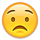• be prepared to deviate from the usual methods and think on your feet.

Oh god no• Hands up who's going to have a nervous breakdown if Q5 is the same as last year? Just me? Seriously though, they've been adding different approaches to the standard questions in the last few years. Wedges are overdue, an overtaking question for 1b is due, a U-tube question for 9 is probably due as well. Don't take anything for granted and be prepared to deviate from the usual methods and think on your feet.
Oh god noIn fairness the stuff you listed there isn't the worst in the world either, U-Tube questions are nice, I was hoping one would appear on my examDon't panic in the exam anyways lads, if you stay calm you will be able to pick up majority of the marks by completing your method

• Also, I've forgotten to post this incredibly valuable piece of advice/motivation. Good luck! http://www.smbc-comics.com/?id=3011

• Daniel2590 wrote: »
Or you can draw a broken line if you want I'm fairly sure

oh yeah that's fine too you just have to differentiate between the force and its components.

• Anyone get k=15?